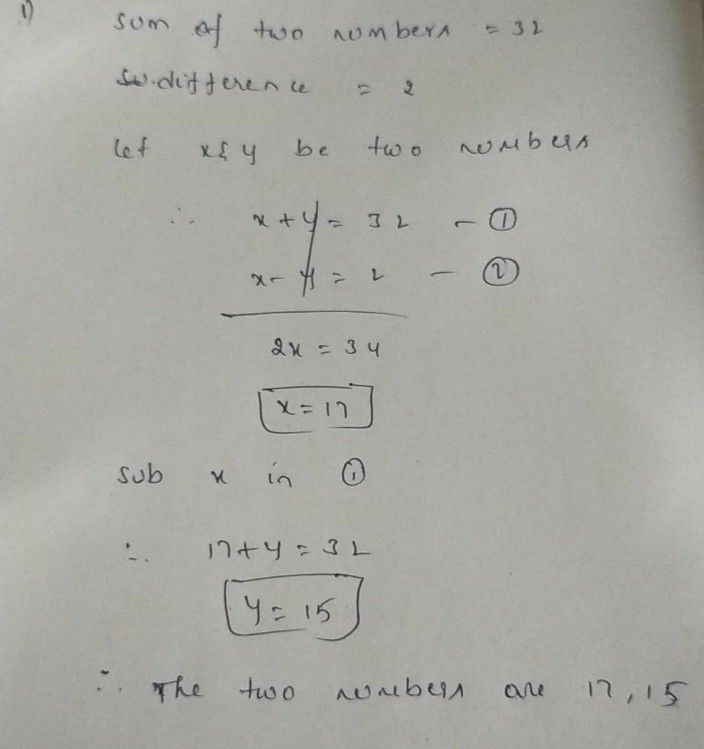Symbol
ProblemLearning $Task2$ $A$ Solve the $systcm9$ of equations by a) graphing b) elimination $c$ substitution B. Solve the problem using any method. The sum of two numbers is $32$ and the difference $1s2$ Find the numbers
7th-9th grade
Other
SolutionQanda teacher - Rohit